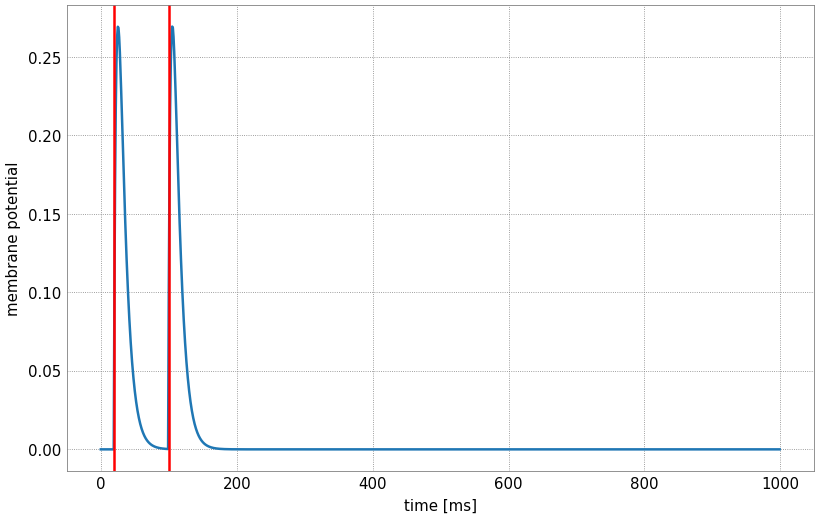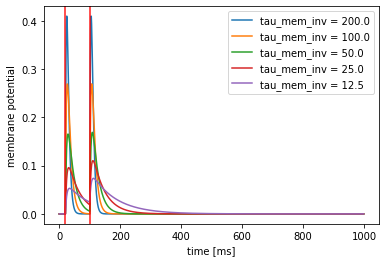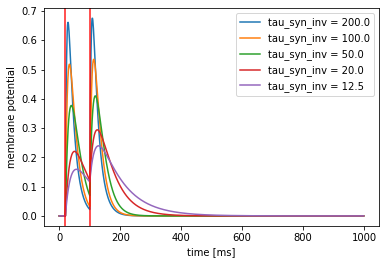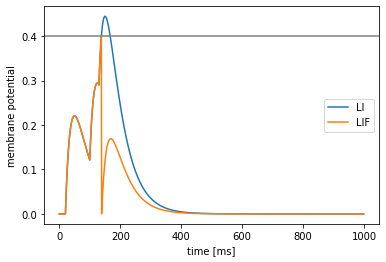# 4. Introduction to spiking systems¶

Artificial neural networks (as we know them from PyTorch) typically consist of linear transformations (such as Convolutions) and point-wise non-linearities such as the infamous rectified linear unit (ReLU). In contrast spiking neural networks take their inspiration from biological neurons. They operate on spikes - events in time - which are combined by linear transformations and integrated by neuron circuits models, such as the LIF neuron model. In other words compared to artificial neural networks, where operation on temporal data is a choice, all spiking neural networks operate on temporal data. One way to look spiking neural networks is to regard them as specific recurrent neural networks (RNNs) with the sequence dimension explicitely identified with time and with the values at each timestep that are exchanged between layers restricted to binary values.

Norse implements state-of-the-art methods to train spiking neural networks in a way that is convenient and accessible to a machine learning researcher. The eventual goal is to fully explore the sparse information processing of spiking neural networks on neuromorphic hardware. But even if you are not motivated by such application and just curious how information processing can be done by systems whose behaviour is closer to that of biological brains, this library might be for you.

1. Simulate neurons

2. Visualize spikes

3. Optimize spiking systems

Note

You can execute the code below by hitting above and pressing Live Code.

## 4.1. Simulating neurons¶

Neurons consist of two things: the activation function and the neuron membrane state. To start working with them, we first have to import Norse:

import norse
norse.__version__

'0.0.7'


### 4.1.1. Defining our first neuron model¶

If you are familiar with machine/deep learning, you recognize the LSTM recurrent neural network, which is applied to a sequence of values. One of the simples neuron models, we have added is the leaky integrator. This particular model integrates an input current and on a “membrane” modelled by a leaky capacitor.

activation = norse.torch.LI()
activation

LI(p=LIParameters(tau_syn_inv=tensor(200.), tau_mem_inv=tensor(100.), v_leak=tensor(0.)), dt=0.001)


### 4.1.2. Defining our input spikes¶

By convention most of our neuron models expect to operate on input spikes. In a time discretised setting this is just a sequence of tensors containing binary values. By default we place spikes on a grid spaced by $$1 \text{ms}$$. So we can define an input spike sequence with spikes at $$20 \text{ms}$$ and $$100 \text{ms}$$ and use the Leaky Integrator (LI) to process it, resulting in a voltage trace.

import torch

data = torch.zeros(1000,1)
data =  1.0
data = 1.0

voltage_trace, _ = activation(data)


### 4.1.3. Visualizing neuron voltage¶

Since this simulation happened over 1000 timesteps (1 second), we expect the model to output the evolution of the membrane potential (the voltage trace), which we can plot:

import matplotlib.pyplot as plt

plt.xlabel('time [ms]')
plt.ylabel('membrane potential')
plt.plot(voltage_trace.detach())
plt.axvline(20, color='red')
plt.axvline(100, color='red')

<matplotlib.lines.Line2D at 0x7ff36e1d5ac0>The time course of the membrane potential for this given input is influenced by the parameters passed to the leaky integrator, we can repeat the experiment with different values. Here we simulate 5 neurons by simply increasing the input dimensionality of the input spike train.

import torch
import norse.torch.module as nm

num_neurons = 5
tau_mem_inv = torch.tensor([200,100,50,25,12.5])
data = torch.zeros(1000,num_neurons)
data =  1.0
data = 1.0

voltage_trace, _ = nm.LI(p=nm.LIParameters(tau_mem_inv=tau_mem_inv, tau_syn_inv=torch.tensor(200)))(data)
plt.xlabel('time [ms]')
plt.ylabel('membrane potential')
for i in range(num_neurons):
plt.plot(voltage_trace.detach()[:,i], label=f'tau_mem_inv = {tau_mem_inv[i]}')
plt.axvline(20, color='red', alpha=0.9)
plt.axvline(100, color='red', alpha=0.9)
plt.legend()

<matplotlib.legend.Legend at 0x7ff36e0f3940>As you can see as the membrane time constant $$\tau_\text{mem}$$ is increased (and consequentely its inverse decreased), the decay of the membrane voltage is slower, but it also reaches a smaller value. The value of the synaptic time constant $$\tau_\text{syn}$$ in turn influences how quickly the synaptic input decays exponentially

num_neurons = 5
tau_syn_inv = torch.tensor([200,100,50,20,12.5])
data = torch.zeros(1000,num_neurons)
data =  1.0
data = 1.0

voltage_trace, _ = nm.LI(p=nm.LIParameters(tau_mem_inv=tau_syn_inv, tau_syn_inv=torch.tensor(50)))(data)
plt.xlabel('time [ms]')
plt.ylabel('membrane potential')
for i in range(num_neurons):
plt.plot(voltage_trace.detach()[:,i], label=f'tau_syn_inv = {tau_syn_inv[i]}')
plt.axvline(20, color='red', alpha=0.9)
plt.axvline(100, color='red', alpha=0.9)
plt.legend()

<matplotlib.legend.Legend at 0x7ff36e0a80d0>## 4.2. Our first Spiking Neuron¶

Spikes that stimulate a neuron in close succession lead to an increasingly higher membrane potential, which is in some sense the foundational principle of information processing with spiking neurons: If one replaces the Leaky Integrator with a Leaky Integrate and Fire neuron model (LIF) one arrives at the simplest spiking neuron model implemented in norse. Since we only return the spike train produced by a LIF neuron by default we define a simple helper function first, which also records the voltage trace. To do so we use the LIFCell module, which only computes the state evolution for one timestep.

def integrate_and_record(cell):
def integrate(input_spike_train):
T = input_spike_train.shape
s = None
spikes = []
voltage_trace = []
for ts in range(T):
z, s = cell(input_spike_train[ts], s)
spikes.append(z)
voltage_trace.append(s.v)
return integrate

v_th = 0.4
cell = nm.LIFCell(p=nm.LIFParameters(tau_mem_inv=torch.tensor(20), tau_syn_inv=torch.tensor(50), v_th=torch.as_tensor(0.4)))
lif_integrate = integrate_and_record(cell)
cell

LIFCell(p=LIFParameters(tau_syn_inv=tensor(50), tau_mem_inv=tensor(20), v_leak=tensor(0.), v_th=tensor(0.4000), v_reset=tensor(0.), method='super', alpha=tensor(100.)), dt=0.001)


By repeating the same experiment, that is stimulating the LI and LIF neuron with spikes at $$20, 100, 130$$ milliseconds, we immediately see the difference: Once the LIF neuron reaches its threshold value $$0.4$$ it fires resulting in an output spike and a reset of its membrane voltage to $$0$$. The output spike train produced by a LIF neuron could be used for further processing by other neurons.

num_neurons = 1
tau_syn_inv = torch.tensor()
data = torch.zeros(1000,num_neurons)
data =  1.0
data = 1.0
data = 1.0

voltage_trace, _ = nm.LI(p=nm.LIParameters(tau_mem_inv=tau_syn_inv, tau_syn_inv=torch.tensor(50)))(data)
zs, lif_voltage_trace = lif_integrate(data)
plt.xlabel('time [ms]')
plt.ylabel('membrane potential')
plt.plot(voltage_trace.detach(), label="LI")
plt.plot(lif_voltage_trace.detach(), label="LIF")
plt.axhline(0.4, color='grey')
plt.legend()

<matplotlib.legend.Legend at 0x7ff36e0b7d60>## 4.3. Encoding Data Into Spikes¶

Now that we have seen how spiking neurons can be simulated in Norse, you might wonder how do you get Spikes in the first place?